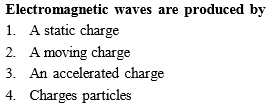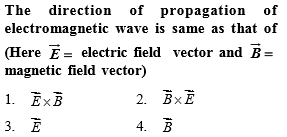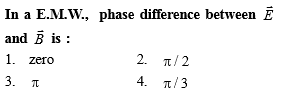• Subject:

Physics

• Topic:

Electromagnetic WavesIn an electromagnetic wave in free space the root mean square value of the electric field is ${E}_{rms}=6$ V/m. The peak value of the magnetic field is

(a) 1.41$×{10}^{-8}T$

(b) $2.83×{10}^{-8}T$

(c) $0.70×{10}^{-8}T$

(d)

NEET

Out of the following options which one can be used to produce a propagating electromagnetic wave?

(a) A stationary charge               (b) A chargeless particle

(c) An accelerating charge          (d) A charge moving at constant velocity

NEET

A radiation of energy 'E' falls normally on a perfectly reflecting surface. The momentum transferred to the surface is (c=velocity of light)

(a)E/c

(b)2E/c

(c)2E/c2

(d)E/c2

NEET

The energy of the EM want is of the order of 15 KeV. To which part of the spectrum does it belong?

(a)X-rays

(b)Infrared rays

(c)Ultraviolet rays

(d)$\gamma$-rays

NEET

Light with an energy flux of 25 x 104 Wm-2 falls on a perfectly reflecting surface at normal incidence. If the surface area is 15cm2 the average force exerted on the surface is

(a)1.25x10-6N

(b)2.50x10-6N

(c)1.20x10-6N

(d)3.0x10-6N

NEET

The condition under which a microwave oven heats up a food item containing water molecules most efficiently in

(a) the frequency of the microwave must match the resonant frequency of the water molecules

(b) the frequency of the microwave  has no relation with natural frequency of water molecules

(c) microwave are heat waves, so always produce heating

(d) infrared waves produce heating in a microwave oven

NEET